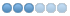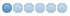# Named parameter #<_ini[axis_2]max_limit> not defined on Programm Start

• blue0cean
• Offline
• Senior Member
•24 Sep 2019 12:26 - 24 Sep 2019 12:34 #146060
Hello Nativcam user,
has one of you an idea why this message when you submit the program the error message:

Named parameter #<_ini[axis_2]max_limit> not defined

Native Cam Version 0.1.14d
Linuxcnc 2.9

appears. It does not matter if I do not give up a task or a task like facing. Here is my ini.

[AXIS_X]
MIN_LIMIT = -10000
MAX_LIMIT = 10000
MAX_VELOCITY = 60
MAX_ACCELERATION = 20

[JOINT_0]
TYPE = LINEAR
HOME = 0.0
HOME_OFFSET = 0.0
HOME_SEQUENCE = 0
VOLATILE_HOME = 0
SCALE = 100
ENC_SCALE = 4096
FERROR = 1
MIN_LIMIT = -10000
MAX_LIMIT = 10000
BACKLASH = 0.000

P = 150.0
I = 0.0
D = 0.0
FF0 = 0.0
FF1 = 0.0
FF2 = 0.0
MAX_OUTPUT = 200.0

[
[AXIS_Z]
MIN_LIMIT = -10000
MAX_LIMIT = 1000
MAX_VELOCITY = 60
MAX_ACCELERATION = 20

[JOINT_2]
TYPE = LINEAR
HOME = 0.0
HOME_OFFSET = 0.0
HOME_SEQUENCE = 0
VOLATILE_HOME = 0
SCALE = 100
ENC_SCALE = 4096
FERROR = 1
MIN_LIMIT = -10000
MAX_LIMIT = 10000
BACKLASH = 0.000

P = 150.0
I = 0.0
D = 0.0
FF0 = 0.0
FF1 = 0.0
FF2 = 0.0
MAX_OUTPUT = 200.0
##### Attachments:
Last edit: 24 Sep 2019 12:34 by blue0cean.

24 Sep 2019 13:42 #146069 by pl7i92
seams you mixed up the valiues and Versions
so for your best guess is to just copy past
[AXIS_X]
MIN_LIMIT = -10000
MAX_LIMIT = 10000
MAX_VELOCITY = 60
MAX_ACCELERATION = 20
to
[AXIS_0]
MIN_LIMIT = -10000
MAX_LIMIT = 10000
MAX_VELOCITY = 60
MAX_ACCELERATION = 20

axis_2 is Z

• blue0cean
• Offline
• Senior Member
•24 Sep 2019 15:09 #146081
Hello pl7i92 thanks for the quick answer.

if I use it like this:
[AXIS_0]
MIN_LIMIT = -10000
MAX_LIMIT = 10000
MAX_VELOCITY = 60
MAX_ACCELERATION = 20
...
[AXIS_2]
MIN_LIMIT = -10000
MAX_LIMIT = 10000
MAX_VELOCITY = 60
MAX_ACCELERATION = 20

comes the message:

LINUXCNC - 2.9.0~pre0
Machine configuration directory is '/home/cnc/linuxcnc/configs/Erna_v1_e Modbus'
Machine configuration file is 'Erna_V1e.ini'
INIFILE=/home/cnc/linuxcnc/configs/Erna_v1_e Modbus/Erna_V1e.ini
VERSION=1.1

check_config: (trivkins kinematics) WARNING:
Unspecified [JOINT_0]MAX_VELOCITY, default used: 1.0
Unspecified [JOINT_0]MAX_ACCELERATION, default used: 1.0
Unspecified [JOINT_1]MAX_VELOCITY, default used: 1.0
Unspecified [JOINT_1]MAX_ACCELERATION, default used: 1.0
Unspecified [JOINT_2]MAX_VELOCITY, default used: 1.0
Unspecified [JOINT_2]MAX_ACCELERATION, default used: 1.0
Unspecified [AXIS_X]MIN_LIMIT, default used: -1e99
Unspecified [AXIS_X]MAX_LIMIT, default used: +1e99
Unspecified [AXIS_Y]MIN_LIMIT, default used: -1e99
Unspecified [AXIS_Y]MAX_LIMIT, default used: +1e99
Unspecified [AXIS_Z]MIN_LIMIT, default used: -1e99
Unspecified [AXIS_Z]MAX_LIMIT, default used: +1e99

check_config: (trivkins kinematics) ERROR:
[JOINT_0]MIN_LIMIT > [AXIS_X]MIN_LIMIT (-10000 > -1e99)
[JOINT_0]MAX_LIMIT < [AXIS_X]MAX_LIMIT (10000 < +1e99)
[JOINT_1]MIN_LIMIT > [AXIS_Y]MIN_LIMIT (-10000 > -1e99)
[JOINT_1]MAX_LIMIT < [AXIS_Y]MAX_LIMIT (10000 < +1e99)
[JOINT_2]MIN_LIMIT > [AXIS_Z]MIN_LIMIT (-10000 > -1e99)
[JOINT_2]MAX_LIMIT < [AXIS_Z]MAX_LIMIT (10000 < +1e99)

check_config validation failed.

24 Sep 2019 15:56 #146086

seams you mixed up the valiues and Versions
so for your best guess is to just copy past
[AXIS_X]
MIN_LIMIT = -10000
MAX_LIMIT = 10000
MAX_VELOCITY = 60
MAX_ACCELERATION = 20
to
[AXIS_0] <<<< this is wrong, there can not be axis 0, just joint 0
MIN_LIMIT = -10000
MAX_LIMIT = 10000
MAX_VELOCITY = 60
MAX_ACCELERATION = 20

axis_2 is Z

• blue0cean
• Offline
• Senior Member
•25 Sep 2019 08:13 #146137
SRY, my mistake.
now I could limit it further, as soon as a tool <> 0 is selected, the error appears. Since the lathe tool table contains no XZ offsets, I have no idea yet.

25 Sep 2019 08:57 #146144

SRY, my mistake.

• zu4lu
• Offline
• New Member
•01 Jan 2021 16:52 #193742 by zu4lu
Did you find what caused this? I have the same problem.
The following user(s) said Thank You: ikkuh

23 May 2021 13:50 #209907 by ikkuh

Did you find what caused this? I have the same problem.

same here now.

Gmoccapy and Probescreen 2. Everything seems to work, only the toollength measurement gives me that error and will not function anymore.

14 Jul 2021 11:07 #214717 by CADdy
The same here...
I don't now where so search.

named parameter #<_ini[axis_2]max_limit> not defined